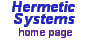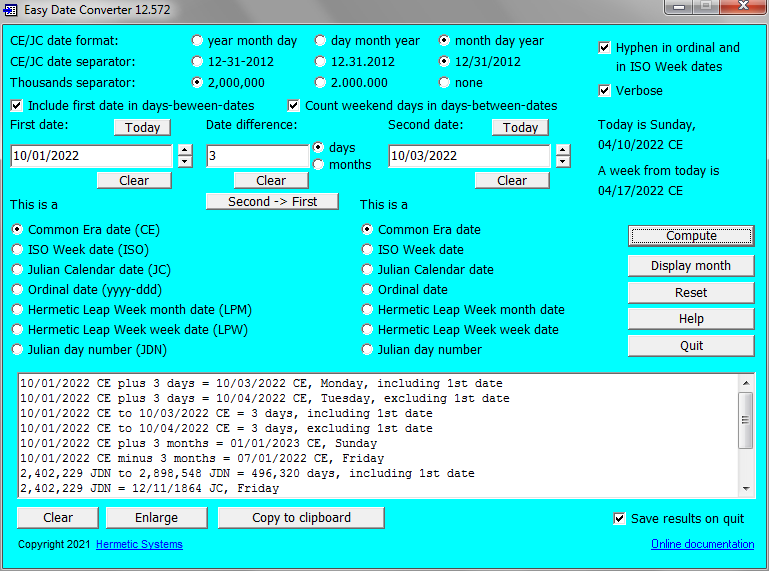# Easy Date Converter## A Multi-Functional Date Conversion and Date Arithmetic Program

How many (week)days between two dates? Should the first day be included in the count? Easy Date Converter allows you to customize your question and get answers easily, and to perform arithmetical operations with Gregorian (a.k.a. Common Era) and Julian dates, dates in the ISO 8601 Week Calendar and in the Hermetic Leap Week Calendar, Julian day numbers and ordinal (day‑in‑year) dates of the form yyyy‑ddd.

Suppose you run an advertisement which expires 45 days from today. What date will that be? How many weekdays are there in 2017? If you have an investment which matures on June 30th, 2019, how many days away is that? What date is the 203rd day of this year? If a loan is made on October 23rd, 2017, for 33 months, when will it be due? When will be (or was) the 10,000th day in your life? How many weekdays in a given year? How many years, months and days until December 21st, 2030? How many business days between two dates? If you need to do this sort of calculation then you need this program.Easy Date Converter provides five calendrical operations:

1. Calculation of the number of days from one date to another (optionally excluding weekend days).
2. Addition of a number of days to a date and subtraction of a number of days from a date.
3. Addition of a number of months to a date and subtraction of a number of months from a date.
4. Interconversion among:
1. Gregorian (a.k.a. Common Era) dates
2. Julian Calendar dates
3. ISO 8601 Week Calendar dates (e.g., 2007-W52-1)